# CSE 203 B Convex Optimization Chapter 9 Unconstrained

• Slides: 23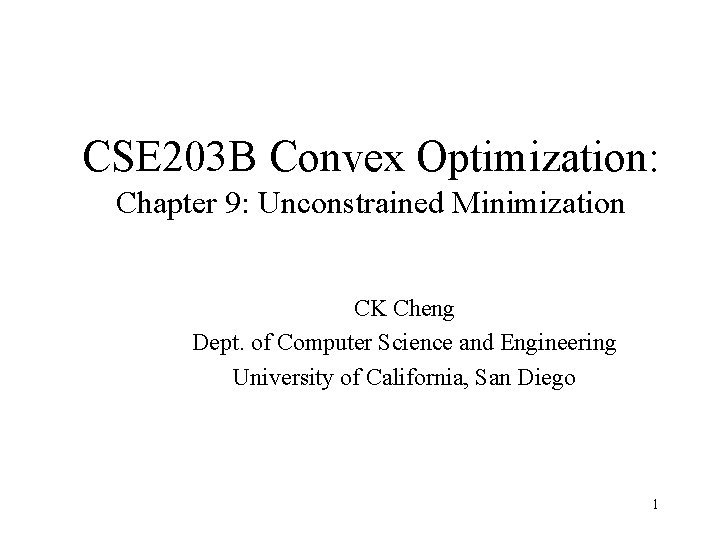CSE 203 B Convex Optimization: Chapter 9: Unconstrained Minimization CK Cheng Dept. of Computer Science and Engineering University of California, San Diego 1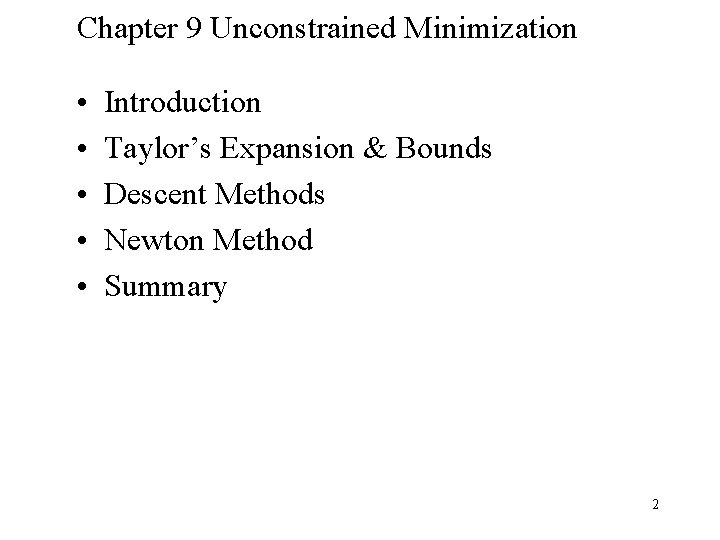Chapter 9 Unconstrained Minimization • • • Introduction Taylor’s Expansion & Bounds Descent Methods Newton Method Summary 2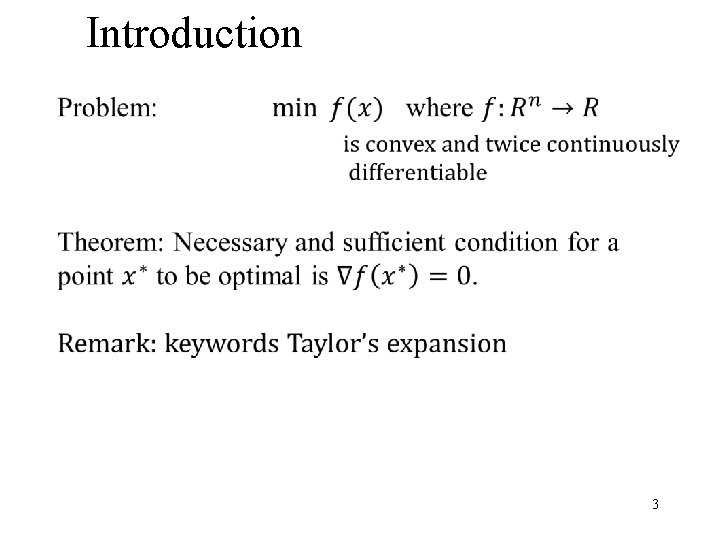Introduction 3Taylor’s Expansion & Bounds: Scalar case 4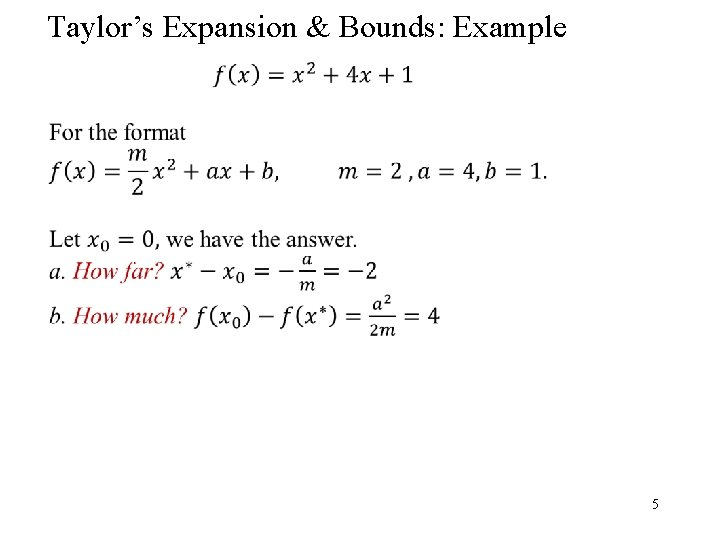Taylor’s Expansion & Bounds: Example 5Taylor’s Expansion & Bounds: Bounds 4 1 3 2 1 6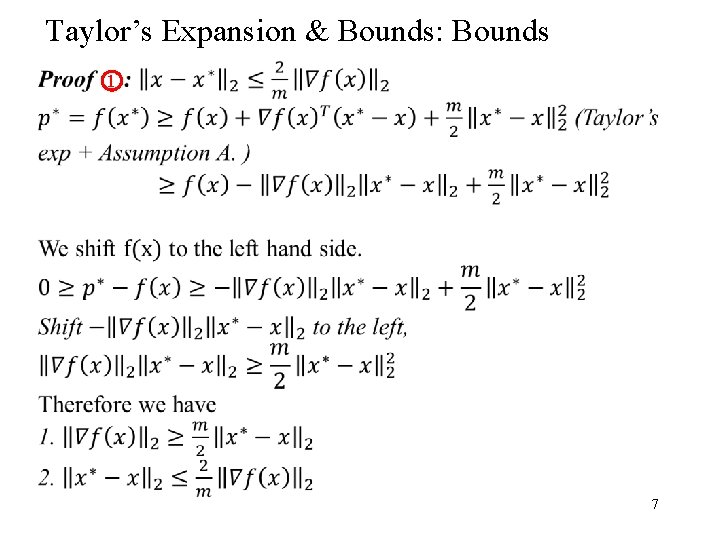Taylor’s Expansion & Bounds: Bounds 1 7Taylor’s Expansion & Bounds: Bounds 2 8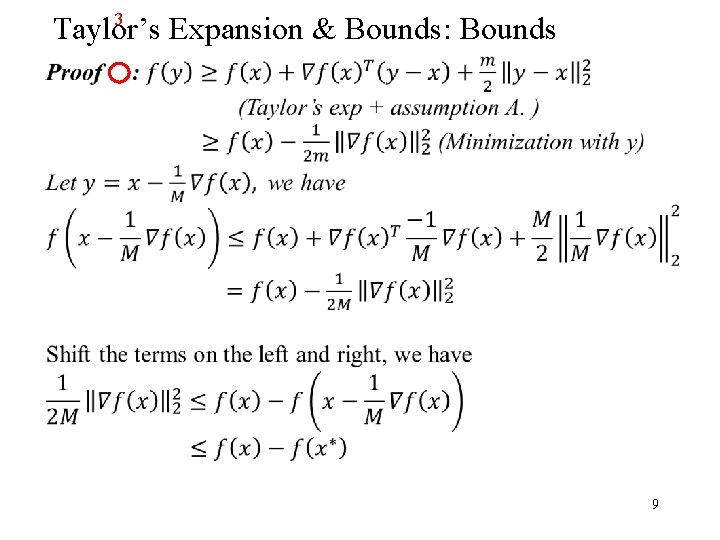3 Taylor’s Expansion & Bounds: Bounds 9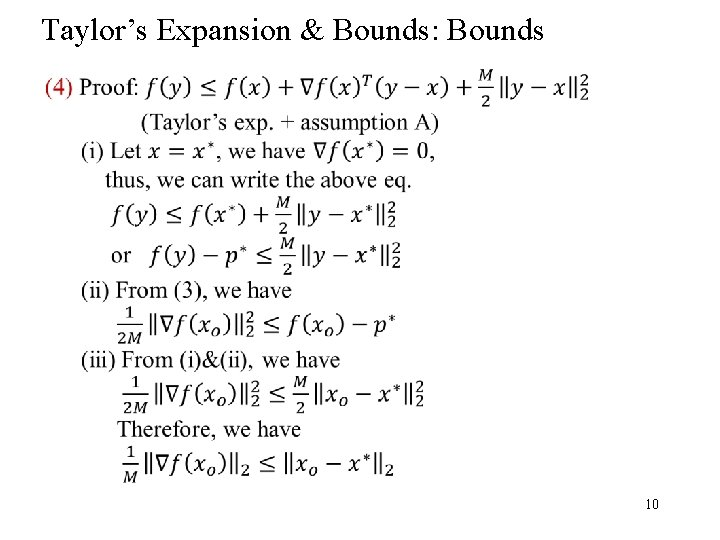Taylor’s Expansion & Bounds: Bounds 10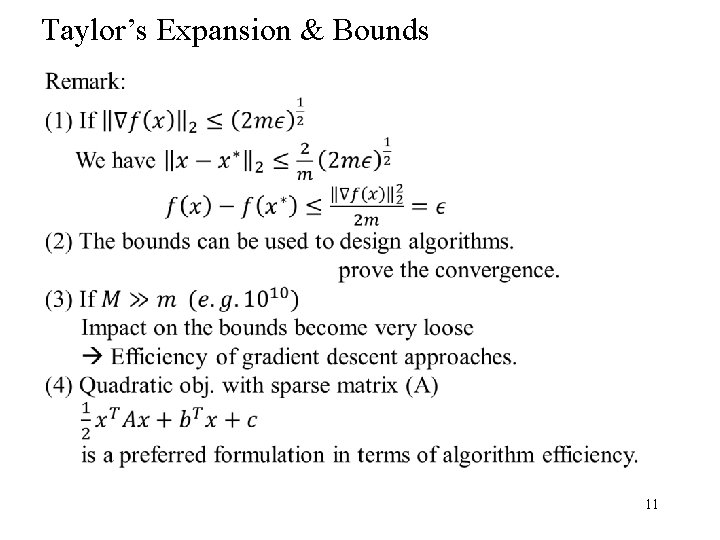Taylor’s Expansion & Bounds 11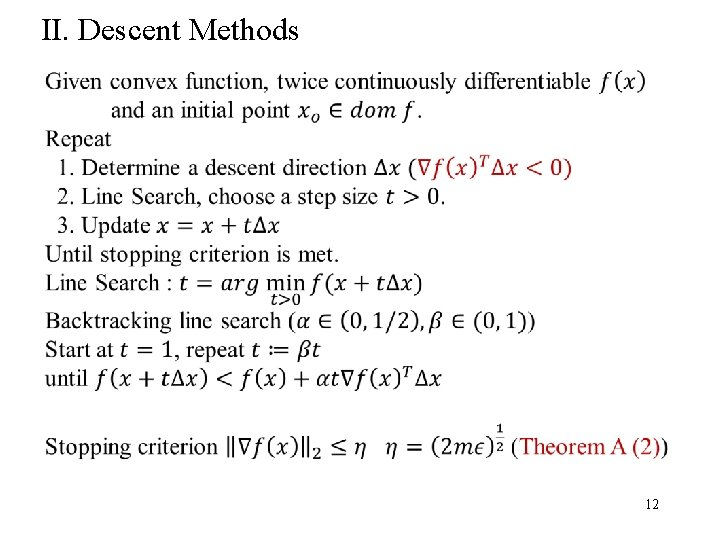II. Descent Methods 12II. Descent Methods: Example 13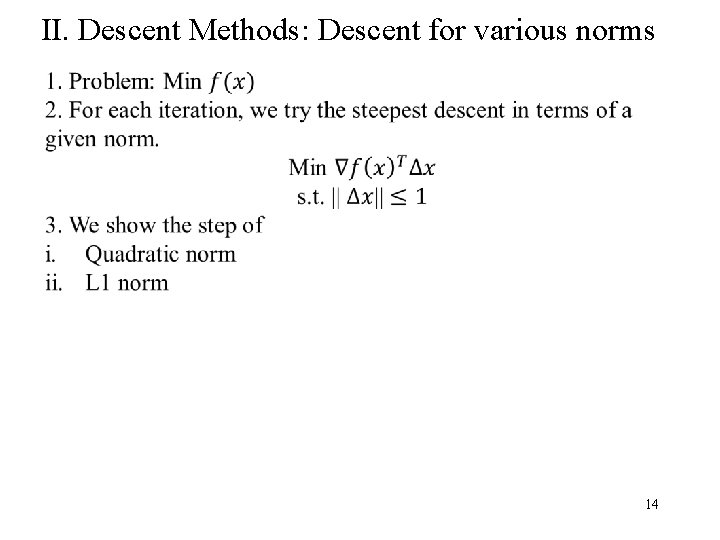II. Descent Methods: Descent for various norms 14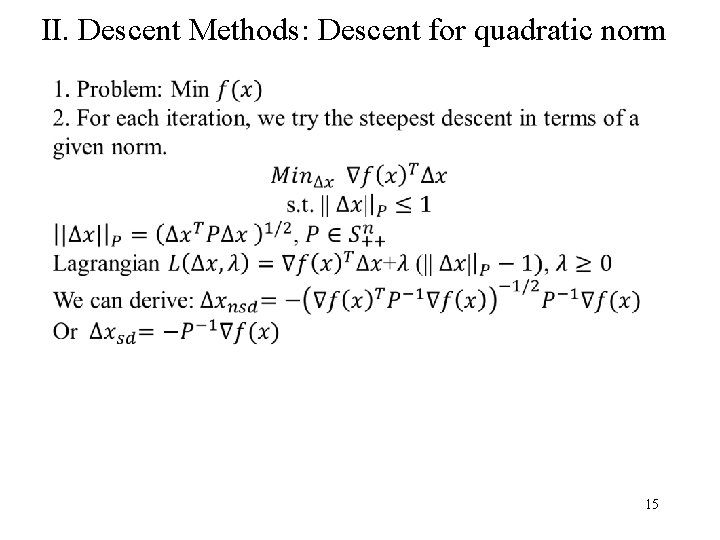II. Descent Methods: Descent for quadratic norm 15II. Descent Methods: Descent for quadratic norm 16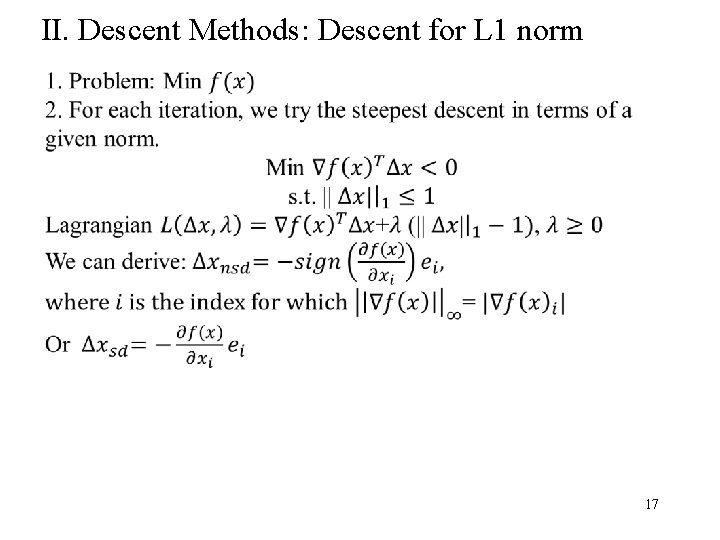II. Descent Methods: Descent for L 1 norm 17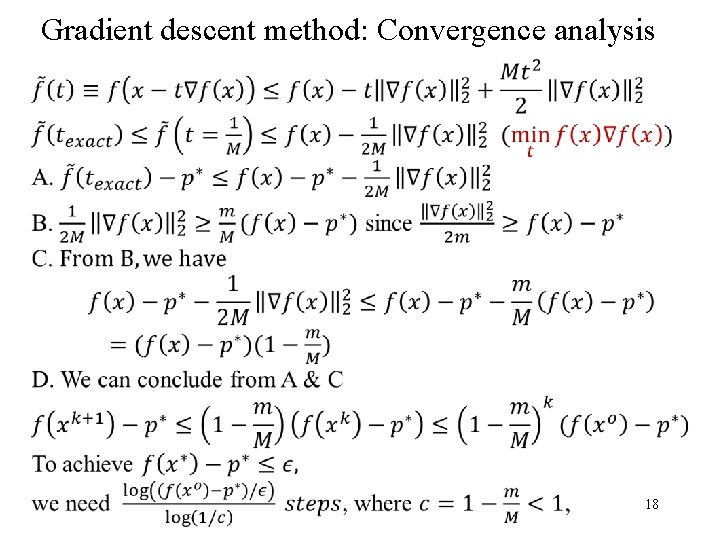Gradient descent method: Convergence analysis 18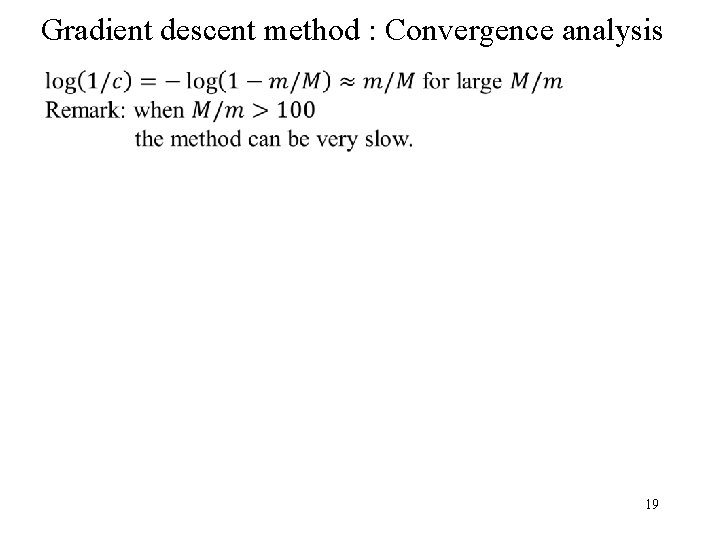Gradient descent method : Convergence analysis 19Newton Step 20Newton Method : Convergence analysis 21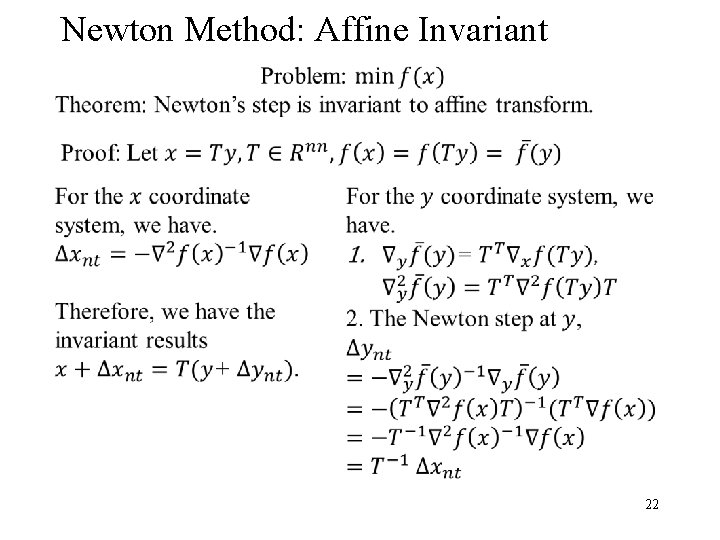Newton Method: Affine Invariant 22Summary 1. Gradient Descent Method: (minimization solution) 1. Vector operations per iteration 2. Linear convergence rate 2. Newton’s Method: (equality solution) 1. Matrix operations per iteration 2. Quadratic convergence rate (near the solution) 3. Gradient Descent Method Variations: 1. Conjugate gradient method 2. Nesterov gradient descent method 3. Quasi-Newton method 23